• MATLAB实现的图像处理基础的案例，如HSI,直方图统计等。
图像处理实验实例作者：@猫西柿

主要说明
MATLAB实现 基础的案例 案例相关参考
HSI/HSV 简单图像增强
imread('d:/911.png');
RGB = ans;
RGB1 = imcrop(RGB,[1000 1000 640 480]);
HSV = rgb2hsv(RGB1);

I=RGB1;
I=double(I);
J=(I-30)*255/70;
row=size(I,1);
col=size(I,2);
for i=1:row
for j=1:col
if(J(i,j)<0)
J(i,j)=0;
end
if J(i,j)>255;
J(i,j)=255;
end
end
end
subplot(2,2,1);imshow(RGB1);subplot(2,2,2);imshow(HSV);
subplot(2,2,3);imshow(RGB2);subplot(2,2,4);imshow(uint8(J));

相关函数介绍:
imreade(url) 读取url路径指定图像（RGB存储）imcrop(rgb,n) 裁剪rgb为n(二维数对)大小imadjust() 参考链接
参考网址:

图像增强——灰度线性、直方图均衡、同态滤波 图像处理——对比度增强 histeq从用法到原理——Matlab直方图均衡化函数

图片裁剪直方图统计/均衡化
实现代码
RGB=imread('d:/911.png');
rgb1=rgb2gray(RGB);
rgb=imresize(rgb1,[240 320]);

[w, h] = size(rgb)
hcount = zeros([1 256]);%直方图统计
for i = 1:w
for j = 1:h
% Accumulation
hcount(rgb(i, j)) = hcount(rgb(i, j)) + 1;
end
end;

figure;

subplot(3,2,2);
imshow(rgb);

subplot(3,2,1);
stem(0:255, hcount,'.');
axis([0 255 0 5000]);

image = rgb%直方图统计
[height, width] = size(image);
NumPixel = zeros(1,256);
for i = 1 : height
for j = 1 : width
k = image(i,j);
NumPixel(k+1) = NumPixel(k+1) + 1;
end
end

%由此开始 直方图均衡化
ProbPixel = zeros(1,256);
for i = 1 : 256
ProbPixel(i) = NumPixel(i) / (height * width);
end

CumPixel = cumsum(ProbPixel);
CumPixel = uint8((256-1) .* CumPixel + 0.5);

outImage = uint8(zeros(height, width));

for i = 1 : height
for j = 1 : width
outImage(i,j) = CumPixel(image(i,j));
end
end

[w, h] = size(outImage);
hcount = zeros([1 256]);
for i = 1:w
for j = 1:h
hcount(outImage(i, j)) = hcount(outImage(i, j)) + 1;
end
end;
%由此开始 直方图均衡化结束

subplot(3,2,3);
stem(0:255, hcount,'.');
axis([0 255 0 5000]);

subplot(3,2,4);
imshow(outImage);

subplot(3,2,5);
imshow(RGB);

相关函数介绍
imresize()函数缩放
参考网址:

直方图 matlab 均值化 直方图 灰度图实现

实现图像的旋转(插值处理)
实现的函数:function im2= MyRotaFun(X,Y)
function im2= MyRotaFun(X,Y)
%函数文件名 MyRotaFun.m

% 读入图片
im = X
% 求出旋转矩阵
a = Y / 180 * pi;
R = [cos(a), -sin(a); sin(a), cos(a)];
R = R'; % 求出旋转矩阵的逆矩阵进行逆向查找
% 计算原图大小
sz = size(im);
h = sz(1);
w = sz(2);
ch = sz(3);
c1 = [h; w] / 2;
% 计算显示完整图像需要的画布大小
hh = floor(w*sin(a)+h*cos(a))+1;
ww = floor(w*cos(a)+h*sin(a))+1;
c2 = [hh; ww] / 2;
% 初始化目标画布
im2 = uint8(ones(hh, ww, 3)*128);
for k = 1:ch
for i = 1:hh
for j = 1:ww
p = [i; j];
pp = (R*(p-c2)+c1);
mn = floor(pp);
ab = pp - mn;
a = ab(1);
b = ab(2);
m = mn(1);
n = mn(2);
% 线性插值方法
if (pp(1) >= 2 && pp(1) <= h-1 && pp(2) >= 2 && pp(2) <= w-1)
im2(i, j, k) = (1-a)*(1-b)*im(m, n, k) + a*(1-b)*im(m+1, n, k)...
+ (1-a)*b*im(m, n, k)     + a*b*im(m, n, k);
end
end
end
end

函数运行主要代码
rgb=imread('你的图像url');
b1 =imresize(rgb,[480 640]);

b15 = imrotate(b1,15);
c15 = MyRotaFun(b1,15);
s15 = imsubtract(b15,c15);

b70 = imrotate(b1,70);
c70 = MyRotaFun(b1,70);
s70 = imsubtract(b70,c70);

subplot(231),imshow(rgb2gray(b15));
subplot(232),imshow(rgb2gray(c15));
subplot(233),imshow(rgb2gray(s15));

subplot(234),imshow(rgb2gray(b70));
subplot(235),imshow(rgb2gray(c70));
subplot(236),imshow(rgb2gray(s70));

参考链接

matlab图像旋转

Edge算子实现(Sobel、apalance)
实验说明：
sobel算子检测边缘log算子检测边缘edeg()库函数检测边缘
sobel自定义函数FunSobel()
function output = FunSobel(input)
img = input;
[H,W]=size(rgb2gray(img));

F=double(img);
U=double(rgb2gray(img));
uSobel=rgb2gray(img);

for i=2:H-1
for j=2:W-1
Gx=(U(i+1,j-1)+2*U(i+1,j)+F(i+1,j+1))-(U(i-1,j-1)+2*U(i-1,j)+F(i-1,j+1));
Gy=(U(i-1,j+1)+2*U(i,j+1)+F(i+1,j+1))-(U(i-1,j-1)+2*U(i,j-1)+F(i+1,j-1));
uSobel(i,j)=sqrt(Gx^2+Gy^2);
end
end
output = im2uint8(uSobel);

end

log自定义函数laplaceFun()
function output = laplaceFun(input)

img_3=mat2gray(input);   %图像矩阵的归一化
[m,n]=size(img_3);
img_4=img_3;       %保留图像的边缘一个像素

L=0;
t=0.2;          %设定阈值

%Laplace算子
for j=2:m-1
for k=2:n-1
L=abs(4*img_3(j,k)-img_3(j-1,k)-img_3(j+1,k)-img_3(j,k+1)-img_3(j,k-1));
if(L > t)
img_4(j,k)=255;  %白
else
img_4(j,k)=0;    %黑
end
end
end

output =img_4;
end

主函数
rgb=imread('你的图像url');
rgb=imresize(rgb,[400,400]);

subplot(2,3,1);imshow(rgb);
subplot(2,3,2);imshow(FunSobel(rgb));%Sobel自定义函数实现
subplot(2,3,3);imshow(laplaceFun(rgb2gray(rgb)));%拉普拉斯自定义实现
subplot(2,3,4);imshow(edge(rgb2gray(rgb),'log'));%库函数边缘log实现
subplot(2,3,5);imshow(edge(rgb2gray(rgb),'sobel'));%库函数边缘sobel实现

参考连接

图像边缘检测——Laplace算子、LOG算子、DOG算子

霍夫变换以及检测直线
霍夫变换的定义

霍夫变化matlab实现 霍夫变换理解

实验流程的思路
获取适当的图形样本对图形样本进行边缘化处理获取二值图像进行霍夫变换寻找霍夫极值并绘制直线
霍夫检测直线实现
%%Hougn变换
I = imresize(I,[480 640]);
J = I;
I = rgb2gray(I);

f=I;
BW=edge(f,'log');

figure;
subplot(2,2,1);
imshow(f);
title('原始图像')

[row,col]=size(BW);
rhomax=round((row*row+col*col)^0.5);
A=zeros(2*rhomax,180);   %这里，实际上rho的取值范围为[-rhomax,rhomax]，
%但是为了后面进行数量统计，转变为[1,2rhomax]
for m=1:row
for n=1:col
if BW(m,n)>0 %判断为边缘
for theta=1:180

r=theta/180*pi; %角度转换
rho=round(m*cos(r)+n*sin(r));
%Hough变换

rho=rho+rhomax+1;
A(rho,theta)=A(rho,theta)+1;
end
end
end
end
[rho,theta]=find(A>150);   %超过n个点视为共线,rho列号，theta行号
nma=length(rho);

subplot(2,2,2);
imshow(J);

for i=1:nma
hold on
m=1:row;
r=theta(i)/180*pi;
n=(rho(i)-rhomax-m*cos(r))/(0.0001+sin(r));
plot(n,m,'blue','LineWidth',1);
end

title('hough自定义实现检测');

[H, T, R] = hough(BW);
P = houghpeaks(H,10);
x = T(P(:, 2));
y = R(P(:, 1));
lines = houghlines(BW, T, R, P);

subplot(2, 2, 3);
imshow(H, [], 'XData', T, 'YData', R, 'InitialMagnification', 'fit'); %hough变换的图像
xlabel('\theta'), ylabel('\rho');
axis on, axis square, hold on;
P = houghpeaks(H, 4); %提取3个极值点
x = T(P(:, 2));
y = R(P(:, 1));
plot(x, y, 's', 'color', 'white'); %标出极值点
lines = houghlines(BW, T, R, P); %提取线段

subplot(2,2,4);imshow(J);hold on;
for k = 1:length(lines)
xy = [lines(k).point1; lines(k).point2];
plot(xy(:, 1), xy(:, 2), 'LineWidth', 2, 'Color', 'yellow');
plot(xy(1, 1), xy(1, 2), 'x', 'LineWidth', 2, 'Color', 'red');
plot(xy(2, 1), xy(2, 2), 'x', 'LineWidth', 2, 'Color', 'blue');
end
title('hough库函数实现');


傅里叶低通滤波
参考网站

傅里叶低通滤波 傅里叶变换

实现代码
img=imread('peppers.png');
img=rgb2gray(img);

subplot(2,2,1);imshow(img);
d0=50;

F=fft2(img);          %傅里叶变换
F1=log(abs(F)+1);   %取模并进行缩放
subplot(2,2,3);imshow(F1,[]);title('傅里叶变换频谱图');

[m n]=size(img);
m_mid=fix(m/2);
n_mid=fix(n/2);
img_f=fftshift(fft2(double(img)));
img_lpf=zeros(m,n);
for i=1:m
for j=1:n
d=sqrt((i-m_mid)^2+(j-n_mid)^2);   %理想低通滤波，求距离
if d<=d0
h(i,j)=1;
else
h(i,j)=0;
end
img_lpf(i,j)=h(i,j)*img_f(i,j);
end
end
img_lpf=ifftshift(img_lpf);
img_lpf=uint8(real(ifft2(img_lpf)));

subplot(2,2,2);imshow(img_lpf);

Fs=fft2(img_lpf);          %傅里叶变换
F2=log(abs(Fs)+1);   %取模并进行缩放
subplot(2,2,4);imshow(F2,[]);title('傅里叶变换频谱图');


形态学处理
参考链接

函数实现 形态学处理

实验流程
图片开（闭）运算处理均值滤波、中值滤波实现
均值滤波 avefilt.m
%均值滤波函数 x是需要滤波的图像,n是模板大小(即n×n)
function d=avefilt(x,n)
a(1:n,1:n)=1;   %a即n×n模板,元素全是1
p=size(x);   %输入图像是p×q的,且p>n,q>n
x1=double(x);
x2=x1;
%A(a:b,c:d)表示A矩阵的第a到b行,第c到d列的所有元素
for i=1:p(1)-n+1
for j=1:p(2)-n+1
c=x1(i:i+(n-1),j:j+(n-1)).*a;  %取出x1中从(i,j)开始的n行n列元素与模板相乘
s=sum(sum(c));                 %求c矩阵(即模板)中各元素之和
x2(i+(n-1)/2,j+(n-1)/2)=s/(n*n); %将模板各元素的均值赋给模板中心位置的元素
end
end
%未被赋值的元素取原值
d=uint8(x2);

中值滤波 midfilt.m
%中值滤波函数。x是需要滤波的图像,n是模板大小(即n×n)
function d=midfilt(x,n)
p=size(x);   %输入图像是p×q的,且p>n,q>n
x1=double(x);
x2=x1;
for i=1:p(1)-n+1
for j=1:p(2)-n+1
c=x1(i:i+(n-1),j:j+(n-1));  %取出x1中从(i,j)开始的n行n列元素,即模板(n×n的)
e=c(1,:);      %是c矩阵的第一行
for u=2:n
e=[e,c(u,:)];     %将c矩阵变为一个行矩阵
end
mm=median(e);      %mm是中值
x2(i+(n-1)/2,j+(n-1)/2)=mm;   %将模板各元素的中值赋给模板中心位置的元素
end
end
%未被赋值的元素取原值
d=uint8(x2);

主要运行函数
clc;
clear all;
close all;
f = rgb2gray(f);
se=strel('square',20);%结构元素
fc=imclose(f,se);%闭运算

fa=avefilt(f,3);
fm=midfilt(f,3);

figure;

subplot(1,3,1);imshow(f);title('原图');
subplot(1,3,2);imshow(fa);title('均值滤波');
subplot(1,3,3);imshow(fm);title('中值滤波');


K-means划分图片
简单理解

图像识别与k-means算法 聚类算法实例：K-Means实现图像分割

图像分割
图像分割即把图像分割成若干不相交的区域，实质是像素的聚类过程，是图像处理的一种方法。可分为：
基于区域技术，如聚类算法基于边缘技术
实现代码
主函数(k-means聚类划分)
clc;
clear all;
close all;
%用k-means算法对图像进行分割
%获得图像大小
[M,N]=size(I);
%获得图像的直方图统计
hist_I=Histeq_Num(I,M,N);

%类别的数量
k=3;
%产生随机数
R=randperm(256);
%初始化中心点
m=R(1:k);
%最大误差
E=0.001;
%直方图的长度
L=length(hist_I);
B=zeros(L,1);
cat_Hist=cat(2,hist_I',B);
m_copy=m;
%开始寻找最佳分割点
flag=1;
while flag
for i=1:L
if cat_Hist(i,1)~=0
%计算每一个灰度级与中心点的欧拉距离
D=abs(m-i);
[C,ind_min]=min(D);
cat_Hist(i,2)=ind_min;
end
end
flag1=0;
for v=1:k
ind=find(cat_Hist(:,2)==v);
sum=0;
num=0;
for j=1:length(ind)
sum=sum+ind(j)*cat_Hist(ind(j));
num=num+cat_Hist(ind(j));
end
m_copy(v)=sum/num;
if abs(m_copy(v)-m(v))<E
flag1=flag1+1;
end
end
if flag1==3
flag=0;
else
m=m_copy;
end
end
m=floor(m);
for i=1:L
if cat_Hist(i,1)~=0
ind=find(I==i);
I(ind)=m(cat_Hist(i,2));
end
end
imshow(I)

Histeq_Num.m直方图统计
%%%直方图计数
function [hist_I]=Histeq_Num(I,M,N)
hist_I=zeros(1,256);
%每一个值加1，消除零值，以与矩阵的下标对应
I=I+1;
%遍历图像，对每一个灰度分量进行数量统计
for i=1:M*N
hist_I(I(i))=hist_I(I(i))+1;
end
end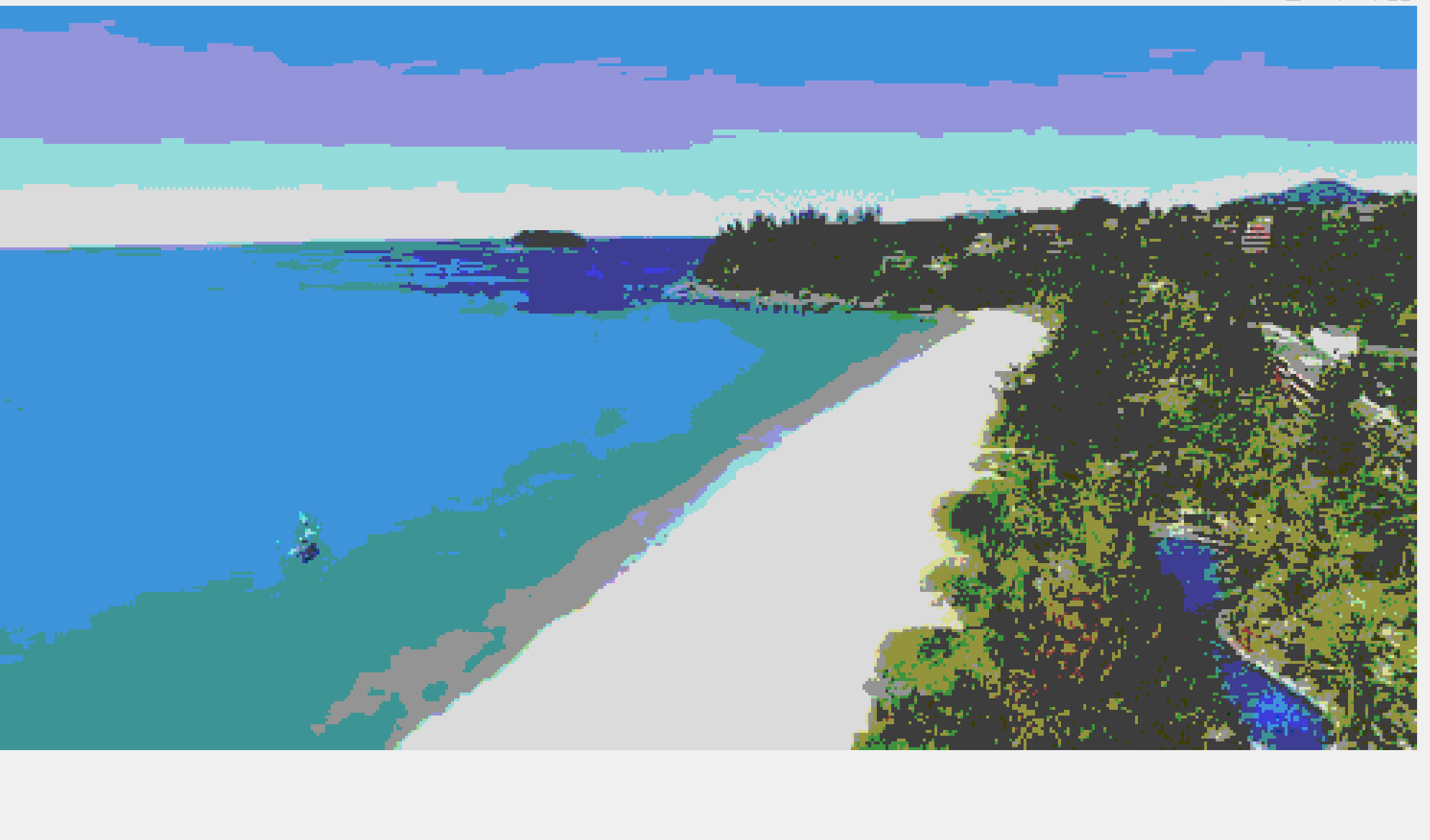展开全文算法 计算机视觉
• ## MATLAB--数字图像处理 图像锐化

万次阅读 多人点赞 2019-12-12 22:40:32
3.于MATLAB环境下编程实现对图片锐化。 三、实验内容     利用图像基于单方向梯度算子，Robert算子，Sobel算 子，Pritt算 子, Laplacian算子，多方向模板，LoG算子编写matlab增强程序;最后，...
一、实验名称
图像锐化
二、实验目的
1.熟悉MATLAB软件的使用。 2.掌握图像锐化的原理及数学运算。 3.于MATLAB环境下编程实现对图片锐化。
三、实验内容
利用图像基于单方向梯度算子，Robert算子，Sobel算 子，Pritt算 子, Laplacian算子，多方向模板，LoG算子编写matlab增强程序;最后，显示边缘图像和锐化增强图像，并对这些仿真结果所呈现的边缘提取和增强效果进行对比分析。
四、实验仪器与设备
Win10 64位电脑 MATLAB2017a
五、实验原理
1.Sobel算子是像素图像边缘检测中最重要的算子之一。在技术上，它是一个离散的一阶差分算子，用来计算图像亮度函数的一阶梯度之近似值。在图像的任何一点使用此算子，将会产生该点对应的梯度矢量或是其法矢量。     2.Roberts边缘检测算子是一种利用局部差分算子寻找边缘的算子，Robert算子图像处理后结果边缘不是很平滑。经分析，由于Robert算子通常会在图像边缘附近的区域内 产生较宽的响应，故采用上述算子检测的边缘图像常需做细化处理，边缘定位的精度不是很高。     3.Prewitt算子是一种一阶微分算子的边缘检测，利用像素点上下、左右邻点的灰度差，在边缘处达到极值检测边缘，去掉部分伪边缘，对噪声具有平滑作用 。其原理是在图像空间利用两个方向模板与图像进行邻域卷积来完成的，这两个方向模板一个检测水平边缘，一个检测垂直边缘。     4. Laplacian算子是n维欧几里得空间中的一个二阶微分算子，定义为梯度grad（）的散度div（）。因此如果f是二阶 可微的实函数，则f的laplacian算子定义为：         (1) f的拉普拉斯算子也是笛卡尔坐标系xi中的所有非混合二阶偏导数求和：         (2) 作为一个二阶 微分算子，拉普拉斯算子把C函数映射到C函数，对于k ≥ 2。
六、实验过程及代码
Sobel算子
 I=imread('a3.jpg'); %读取图像
I1=rgb2gray(I); %将彩色图变成灰色图
subplot(131),imshow(I1),title('原图');

model=[-1,0,1;
-2,0,2;
-1,0,1];
[m,n]=size(I1);
I2=double(I1);

for i=2:m-1
for j=2:n-1
I2(i,j)=I1(i+1,j+1)+2*I1(i+1,j)+I1(i+1,j-1)-I1(i-1,j+1)-2*I1(i-1,j)-I1(i-1,j-1);
end
end
subplot(132),
imshow(I2),title('边缘提取后的图像');
I2 = I2 + double(I1);
subplot(133),
imshow(uint8(I2)),title('锐化后的图像');

Robert算子
 I=imread('a3.jpg'); %读取图像
I1=rgb2gray(I); %将彩色图变成灰色图
subplot(131),imshow(I1),title('原图');
model=[0,-1;1,0];
[m,n]=size(I1);
I2=double(I1);
for i=2:m-1
for j=2:n-1
I2(i,j)=I1(i+1,j)-I1(i,j+1);
end
end
subplot(132),imshow(I2),title('边缘提取后的图像');
I2 = I2 + double(I1);

subplot(133),
imshow(uint8(I2)),title('锐化后的图像');

Prewitt算子
 I=imread('a3.jpg');
I1=rgb2gray(I); %将彩色图变成灰色图
subplot(131);
imshow(I1),title('原图');
model=[-1,0,1;
-1,0,1;
-1,0,1];
[m,n]=size(I1);
I2=I1;
for i=2:m-1
for j=2:n-1
tem=I1(i-1:i+1,j-1:j+1);
tem=double(tem).*model;

I2(i,j)=sum(sum(tem));
end
end
subplot(132),
imshow(uint8(I2)),title('边缘提取后的图像');
I2=I2+I1;
subplot(133),
imshow(I2),title('图像锐化')

laplacian算子
I=imread('a3.jpg');
I1=mat2gray(I);%实现图像矩阵的归一化操作
[m,n]=size(I1);
newGrayPic=I1;%为保留图像的边缘一个像素
LaplacianNum=0;%经Laplacian算子计算得到的每个像素的值
LaplacianThreshold=0.2;%设定阈值
for j=2:m-1 %进行边界提取
for k=2:n-1
LaplacianNum=abs(4*I1(j,k)-I1(j-1,k)-I1(j+1,k)-I1(j,k+1)-I1(j,k-1));
if(LaplacianNum > LaplacianThreshold)
newGrayPic(j,k)=255;
else
newGrayPic(j,k)=0;
end
end
end
I2=rgb2gray(I); %将彩色图变成灰色图
subplot(131),imshow(I2),title('原图');
subplot(132),
imshow(newGrayPic);
title('Laplacian算子的处理结果')
t=I1+newGrayPic;
subplot(133),imshow(t),title('图像锐化后')

Log算子
 t=imread('a3.jpg');
t=rgb2gray(t);
[m,n]=size(t);
subplot(1,3,1),imshow(t),title('原图')
tt=t;
model=[0,0,1,0,0;
0,1,2,1,0;
1,2,-16,2,1;
0,0,1,0,0;
0,1,2,1,0;]

for i=3:m-2
for j=3:n-2
tem=double(t(i-2:i+2,j-2:j+2)).*model;
x=sum(sum(tem));
tt(i,j)=x;
end
end

subplot(1,3,2),imshow(tt),title('log提取边缘后')
t_1=double(tt)+double(t);
subplot(1,3,3),imshow(uint8(t_1)),title('log锐化后')

七、实验结果与分析
Sobel算子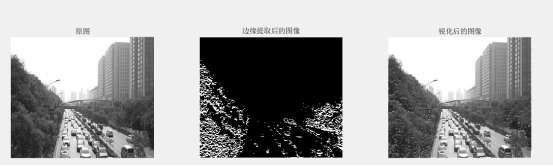Robert算子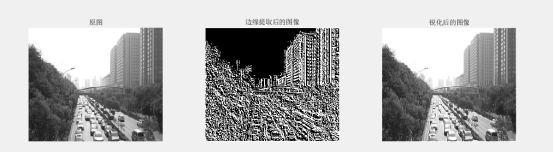Prewitt算子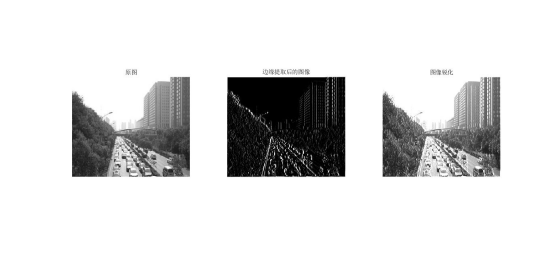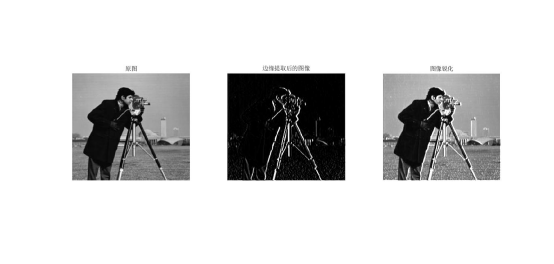laplacian算子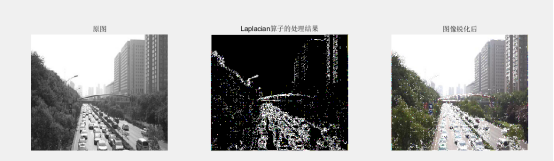Log算子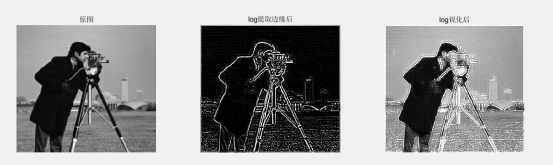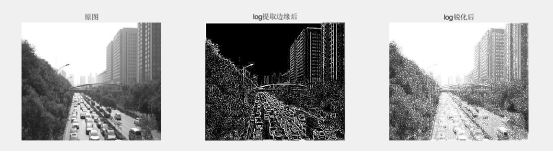总结     图像锐化(image sharpening)是补偿图像的轮廓，增强图像的边缘及灰度跳变的部分，使图像变得清晰，分为空间域处理和频域处理两类。图像锐化是为了突出图像上地物的边缘、轮廓，或某些线性目标要素的特征。这种滤波方法提高了地物边缘与周围像元之间的反差，因此也被称为边缘增强。我的理解：图像锐化就是通过使得图像的边缘更加突出，可以是原图与原图的高频部分叠加而成。     Sobel算法与Priwitt算法的思路相同，属于同一类型，因此处理效果基本相同。Roberts算法的模板为2*2，提取信息较弱。单方向锐化经过处理之后，也可以对边界进行增强。Laplacian算子对噪声比较敏感，Laplacian算子有一个缺点是它对图像中的某些边缘产生双重响应。所以图像一般先经过平滑处理，通常把Laplacian算子和平滑算子结合起来生成一个新的模板-Log算子。
八、实验总结及心得体会
通过此次实验，自己学习了图像锐化的几种算子。开始的时候，不懂每个算子的来源，后面看了老师的推导过程后，发现其实锐化就是先对图像进行差分（类似求导），再叠加到原图上。这里主要的问题就是对算子的差分原理的验证，然后在MATLAB上机过程中，没有注意到数据类型的限制，导致数据上溢。
更多
获取更多资料、代码，微信公众号：海轰Pro 回复 海轰 即可
展开全文图像锐化
• 基于MATLAB图像处理设计题目 图片叠加。设计要求 将一幅礼花图片和一幅夜景图片做叠加运算，使达到烟花夜景的美图效果。设计方案3.1、设计思路 利用matlab强大的图像处理功能，通过编写程序，实现对两幅图片的像素...

基于MATLAB图像处理
设计题目
图片叠加。
设计要求
将一幅礼花图片和一幅夜景图片做叠加运算，使达到烟花夜景的美图效果。
设计方案
3.1、设计思路
利用matlab强大的图像处理功能，通过编写程序，实现对两幅图片的像素进行线性运算，利用灰度变换的算法使图片达到预期的效果。
3.2、软件介绍
MATLAB是matrix&laboratory两个词的组合，意为矩阵工厂（矩阵实验室）。是由美国mathworks公司发布的主要面对科学计算、可视化以及交互式程序设计的高科技计算环境。它将数值分析、矩阵计算、科学数据可视化以及非线性动态系统的建模和仿真等诸多强大功能集成在一个易于使用的视窗环境中，为科学研究、工程设计以及必须进行有效数值计算的众多科学领域提供了一种全面的解决方案，并在很大程度上摆脱了传统非交互式程序设计语言（如C、Fortran）的编辑模式，代表了当今国际科学计算软件的先进水平。
MATLAB和Mathematica、Maple并称为三大数学软件。它在数学类科技应用软件中在数值计算方面首屈一指。MATLAB可以进行矩阵运算、绘制函数和数据、实现算法、创建用户界面、连接其他编程语言的程序等，主要应用于工程计算、控制设计、信号处理与通讯、图像处理、信号检测、金融建模设计与分析等领域。
MATLAB的基本数据单位是矩阵，它的指令表达式与数学、工程中常用的形式十分相似，故用MATLAB来解算问题要比用C，FORTRAN等语言完成相同的事情简捷得多，并且MATLAB也吸收了像Maple等软件的优点，使MATLAB成为一个强大的数学软件。在新的版本中也加入了对C，FORTRAN，C++，JAVA的支持。可以直接调用，用户也可以将自己编写的实用程序导入到MATLAB函数库中方便自己以后调用，此外许多的MATLAB爱好者都编写了一些经典的程序，用户直接进行下载就可以用。
3.3、常见简单程序语句及算法分析
3.4、图片叠加及灰度变换分析
程序1
[m,n,l]=size(C);
for i=1:m
for j=1:n
for k=1:l
D(i,j,k)=C(i,j,k)+ B(i,j,k);
end
end
end
此程序的主要功能是对两幅图片通过算法来实现叠加的效果，程序中的几个变量都是像素点的值，通过三个循环使得两幅图片对应的值线性相加，最大值应该是以255输出，超过255也是以255输出。
程序2
J = imadjust(I,[low_in high_in], [low_out high_out])
四、设计步骤
（1）处理之前，我们先来看看两幅原图，一幅是带有礼花的图片，另一幅是一幅东方明珠的夜景图。图 1图 2
通过图片我们发现，礼花的图片非常的亮，而夜景图则显得有些灰暗。我们推测，如果让礼花和夜景的图片叠加到一起，会不会由于礼花的亮度太大而掩盖了城市的夜光，达不到我们想要的效果。
（2）打开matlab图像处理软件，7.0及以上版本就可以，打开之后，显示界面如下（我的版本是2012b）。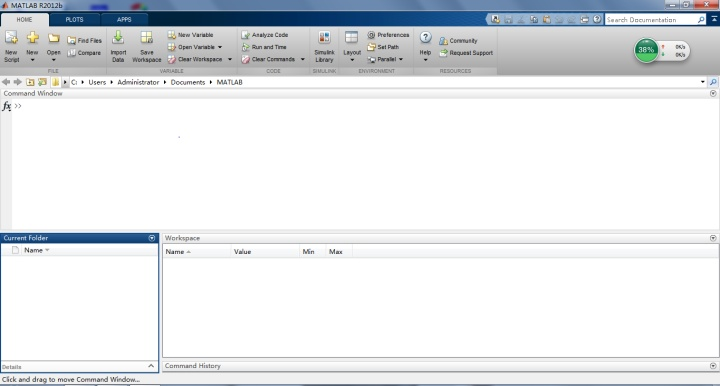图3
新建script文件，点击’New script‘，或点击’New‘，选择script。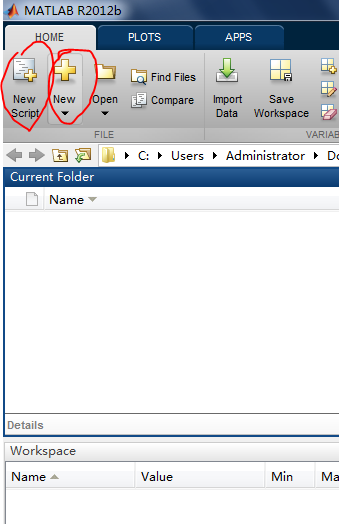图4
（4）在打开的界面之中输入程序。图片的位置是你所要用的图片的准确位置，尽量写详细点，减少计算机的读取时间，加快读取速度。如图所示。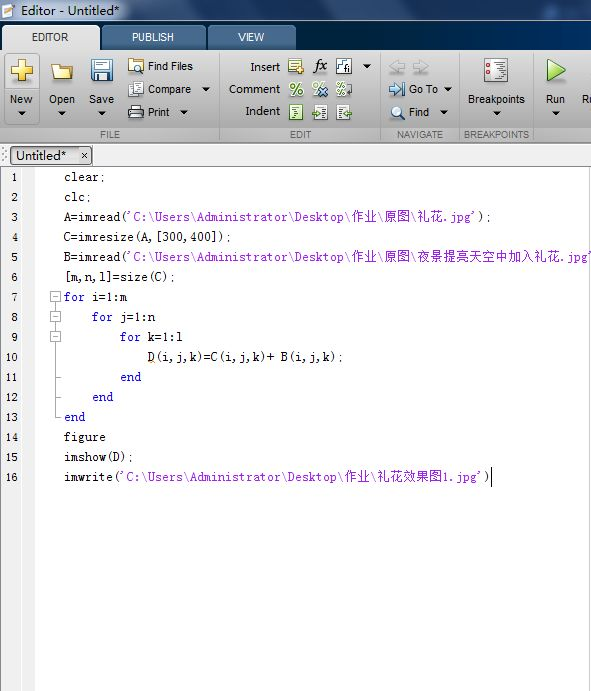图5
（5）点击运行按钮，即界面上的绿色按钮。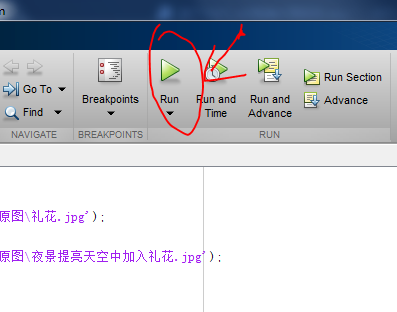图6
（6）查看效果，如下图。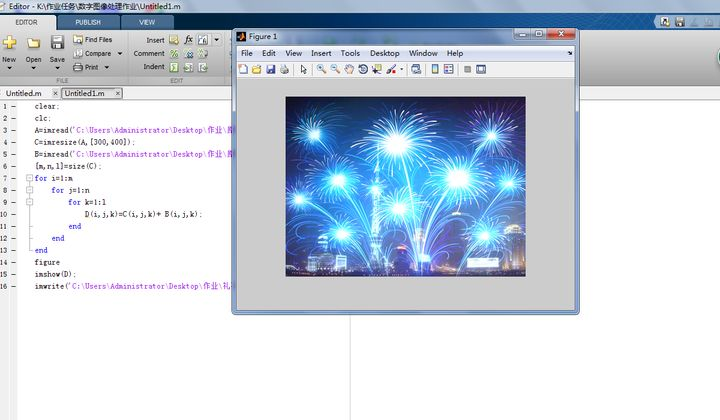图7
通过处理后的图片，我们看到由于礼花太亮，完全将城市的夜景掩盖住了，效果不是太理想。我们设想加入灰度变换函数，将礼花的图片变的暗一点，将夜景的亮度提高一点，看看效果怎么样，加入灰度变换程序，如下图。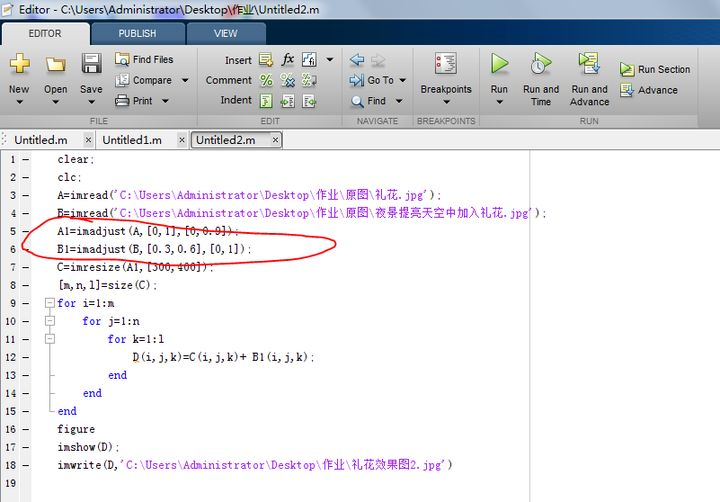图8
（7）软件调试后运行程序，如下图。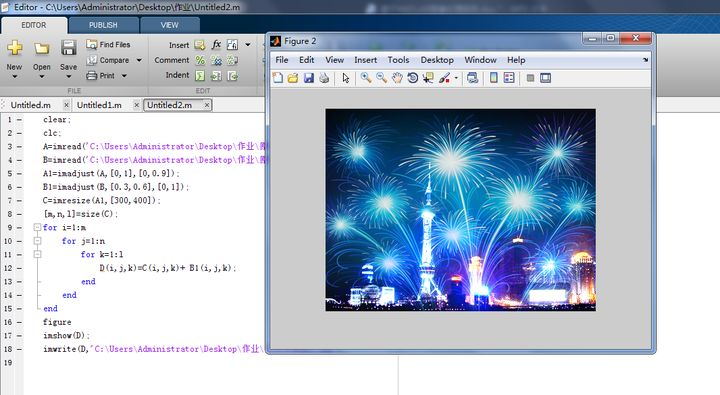图9
结果显示加入灰度变换的函数之后，图片效果较之前好多了，达到了预期的目的。
五、软件代码
通过matlab进行图像处理，代码如下：
clear;
clc;
C=imresize(A,[300,400]);
[m,n,l]=size(C);
for i=1:m
for j=1:n
for k=1:l
D(i,j,k)=C(i,j,k)+ B(i,j,k);
end
end
end
figure
imshow(D);
调试之后的程序代码如下：
clear;
clc;
C=imresize(A1,[300,400]);
[m,n,l]=size(C);
for i=1:m
for j=1:n
for k=1:l
D(i,j,k)=C(i,j,k)+ B1(i,j,k);
end
end
end
figure
imshow(D);
处理后的图片如下：图 10

六、结果分析
通过两幅图的对比，发现第二幅图片较第一幅，效果明显增强。是由于加入灰度变换函数，使原图的灰度值发生变化，以达到实际的效果。效果对比图11
心得体会
通过这次任务，以前在课堂上没太听明白怎么回事的东西，通过上网，查资料，以及用软件处理，通通实践了一遍，加深了对这门课程的认识和理解。Matlab是一款功能很强大的应用软件，它不仅可以对图像进行处理，而且可以进行各种数字计算和符号计算功能，具有绘图功能，语言体系等等。这次的任务我们乐在其中，喜悦的是看到了成果，内心充满了满足感和成就感，就如同看到了图片中的烟花，有种过年的感觉。不过这些都只是皮毛而已，要想真正地掌握它，还得更进一步地学习理论知识。
参考文献：
【冈萨雷斯 数字图像处理（MATLAB版）】[美] RafaelC.Gonzalez RichardE.Woods StevenL.Eddins 著 电子工业出版社。
【数字图像处理及MATLAB实现】杨杰 主编 电子工业出版社。


展开全文算法 可视化 python java 人工智能
• 掌握matlab环境下，函数的编写方法；熟悉并了解图像处理中空域滤波的概念，通过实际操作掌握滤波器的生成和基本的滤波；理解图像锐化的实现方法和步骤，理解图像锐化的基本理论。

Matlab图像处理创新实践-实验1【图像滤波基础（1）】Matlab图像处理创新实践-实验2【图像滤波基础（2）】Matlab图像处理创新实践-实验3【图像锐化】Matlab图像处理创新实践-实验4【大实验——GUI综合设计】
Matlab实验4——GUI程序操作说明

目   录
一、实验目的
二、实验内容
三、实验过程及结果
步骤1、2
步骤3
步骤4
步骤5
步骤6、7
步骤8、9、10
四、分析与思考
附录：代码汇总
主文件——ex3.m
函数文件

一、实验目的

掌握matlab环境下，函数的编写方法；熟悉并了解图像处理中空域滤波的概念，通过实际操作掌握滤波器的生成和基本的滤波；理解图像锐化的实现方法和步骤，理解图像锐化的基本理论。

二、实验内容

步骤1：用手机给自己拍一张美照（必须是本人照片）；
步骤2：将你的照片放到计算机内；
步骤 4：利用rgb2gray()函数将图像转换为灰度图像；
步骤5：利用一种平滑滤波器，F，如9*9的均值滤波器或高斯滤波器，通过new_im = filter2(F, im)对图像进行滤波；
步骤6：利用double()将原图像类型转换为浮点型，然后按照“原图像 + 原图像 - 平滑图像”的模式，进行计算；
步骤7：利用uint8()或mat2gray()将得到的结果图像，进行类型转换，并将原图像和锐化后的图像一同显示在窗口中；
步骤8：利用一种边缘滤波器，FF，如sobel、prewitt等，通过new_im = filter2(FF, im)对图像进行滤波；
步骤9：利用double()将原图像类型转换为浮点型，然后按照“原图像 + 边缘图像”的模式，进行计算；
步骤10：利用uint8()或mat2gray()将得到的结果图像，进行类型转换，并将原图像和锐化后的图像一同显示在窗口中；
注意：锐化操作的代码需形成函数文件（.m后缀），文件名为姓名的汉语拼音，如zhangsan.m，参考原型如下：
function out_im = zhangsan(input_im)

三、实验过程及结果

【在这里描述下你的实验操作过程和相应代码，以及你得到的最终结果，需图文并茂】

步骤1、2

步骤1：用手机给自己拍一张美照（必须是本人照片）；
步骤2：将你的照片放到计算机内；
Matlab内置图片：Peppers.png

步骤3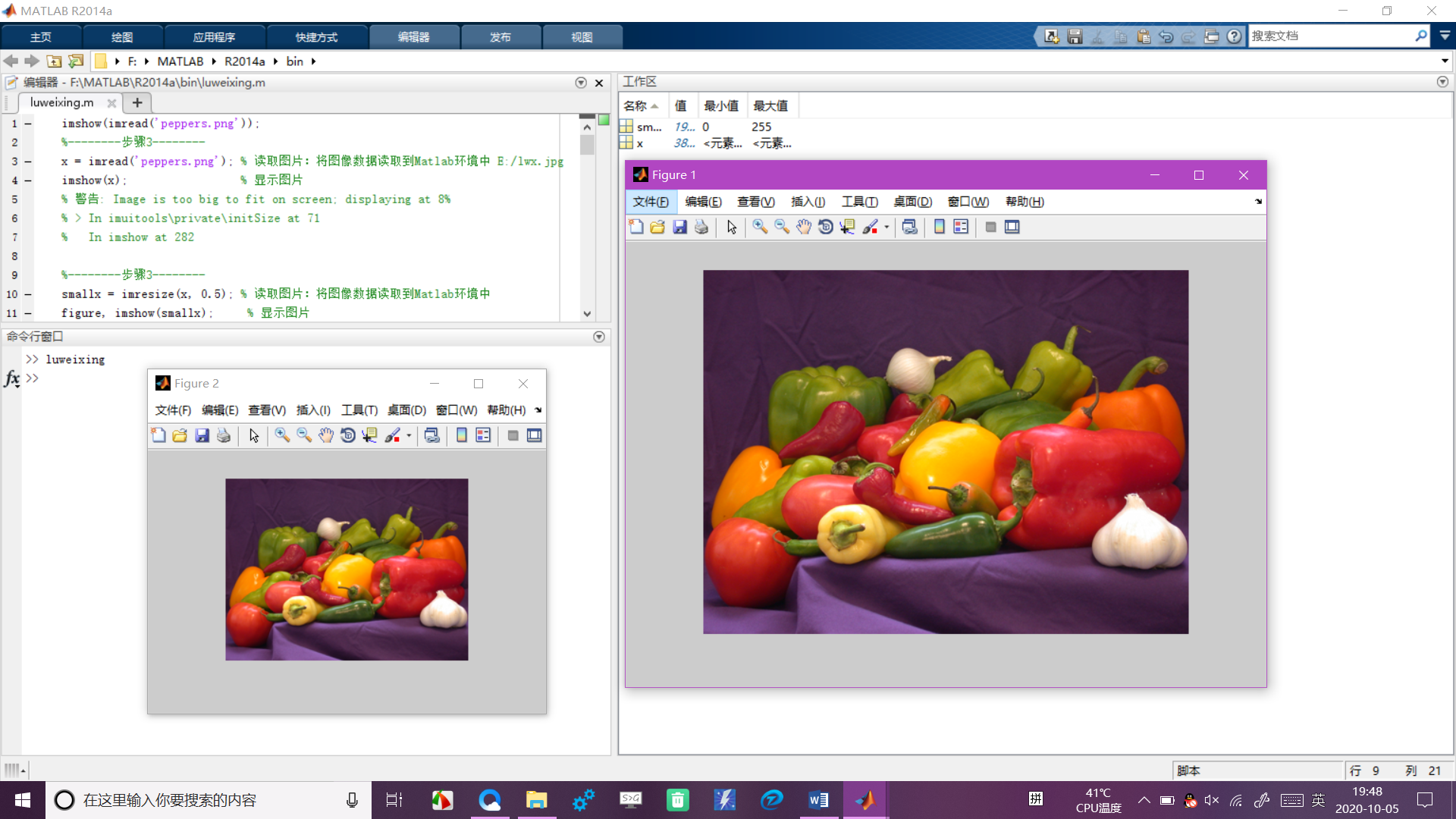步骤4

步骤 4：利用rgb2gray()函数将图像转换为灰度图像；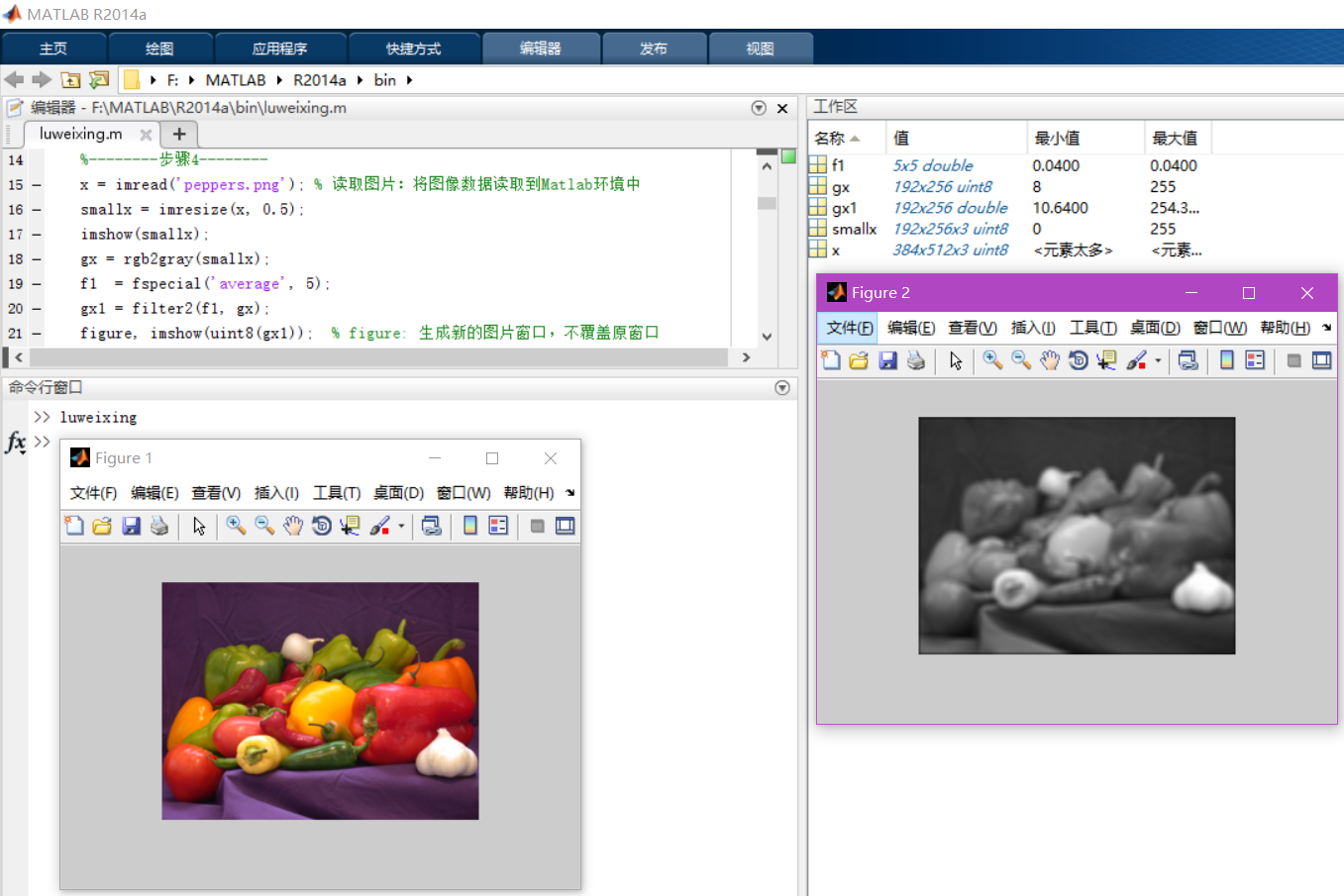步骤5

步骤5：利用一种平滑滤波器，F，如9*9的均值滤波器或高斯滤波器，通过new_im = filter2(F, im)对图像进行滤波；
Matlab内置图片：saturn.png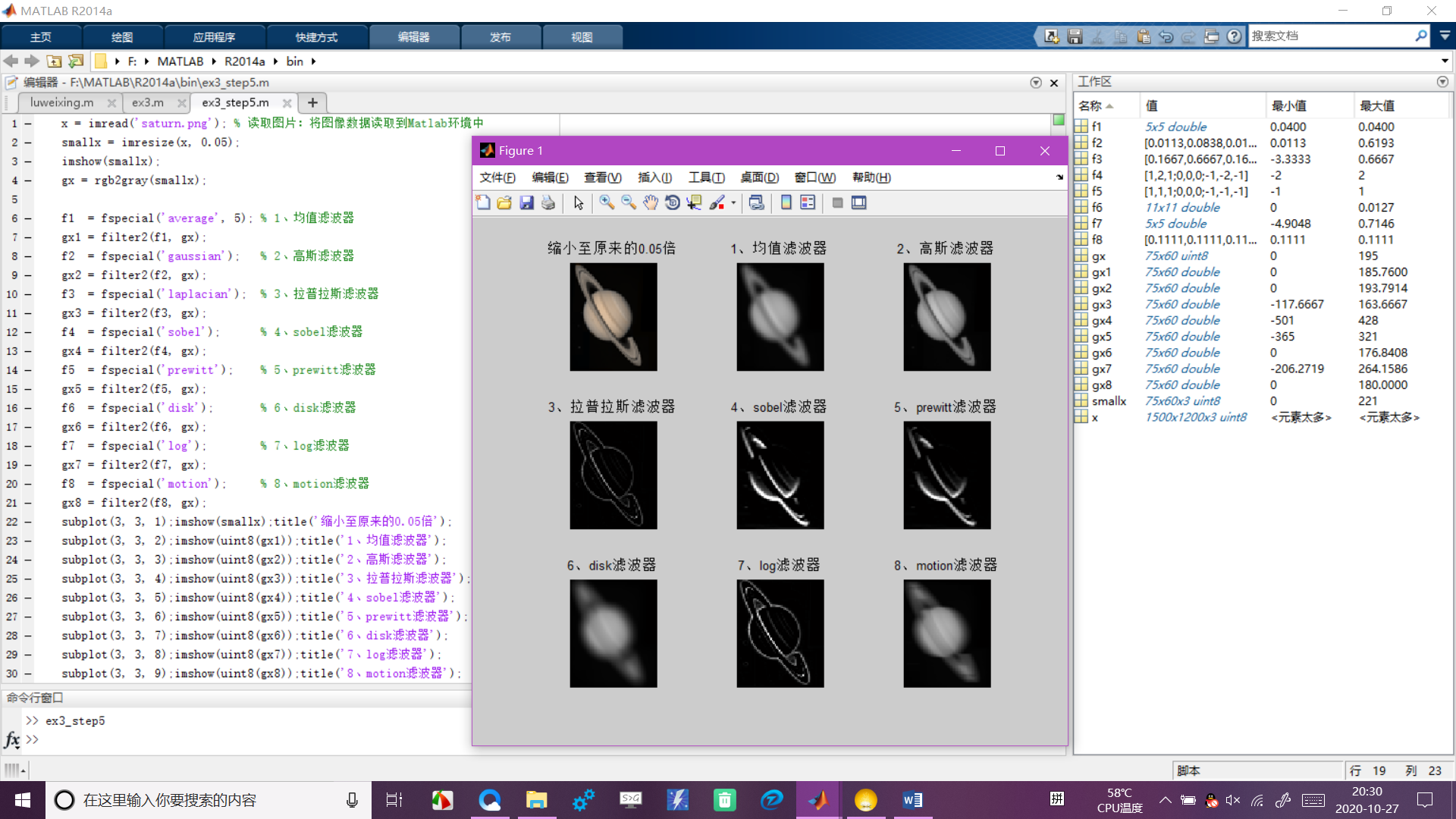步骤6、7

步骤6：利用double()将原图像类型转换为浮点型，然后按照“原图像 + 原图像 - 平滑图像”的模式，进行计算；
步骤7：利用uint8()或mat2gray()将得到的结果图像，进行类型转换，并将原图像和锐化后的图像一同显示在窗口中；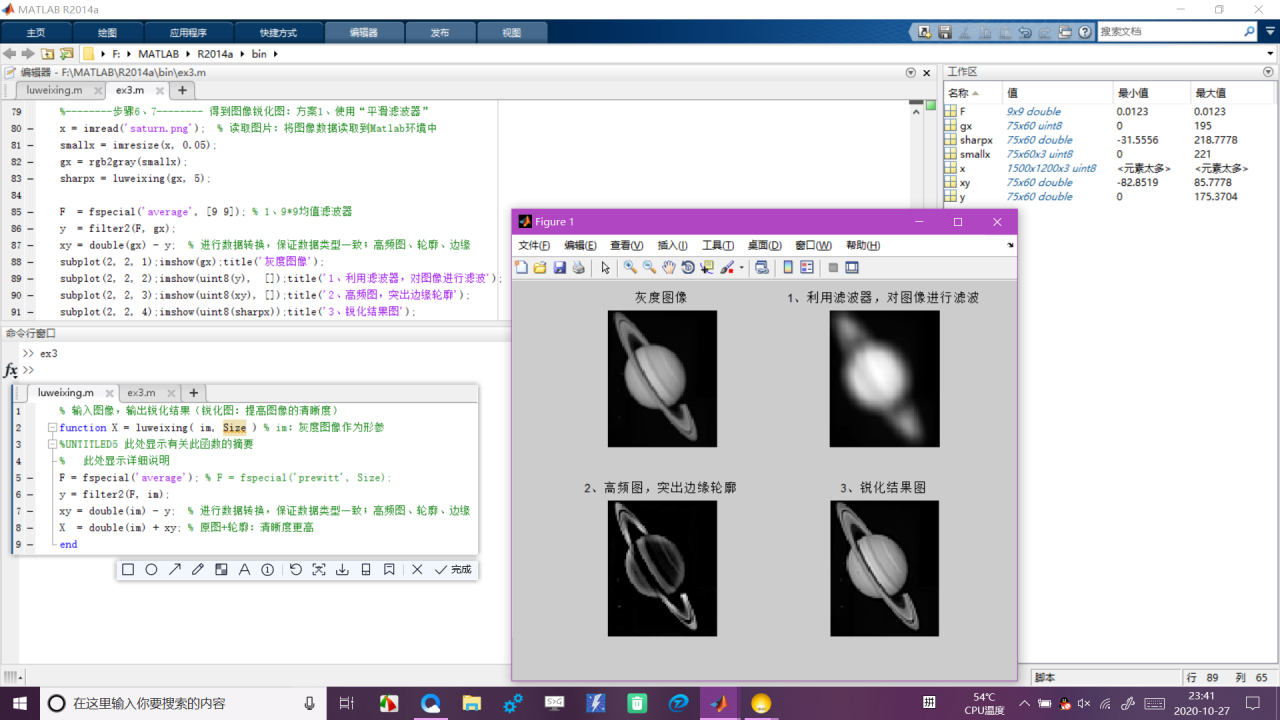步骤8、9、10

步骤8：利用一种边缘滤波器，FF，如sobel、prewitt等，通过new_im = filter2(FF, im)对图像进行滤波；
步骤9：利用double()将原图像类型转换为浮点型，然后按照“原图像 + 边缘图像”的模式，进行计算；
步骤10：利用uint8()或mat2gray()将得到的结果图像，进行类型转换，并将原图像和锐化后的图像一同显示在窗口中；
注意：锐化操作的代码需形成函数文件（.m后缀），文件名为姓名的汉语拼音，如zhangsan.m，参考原型如下：
function out_im = zhangsan(input_im)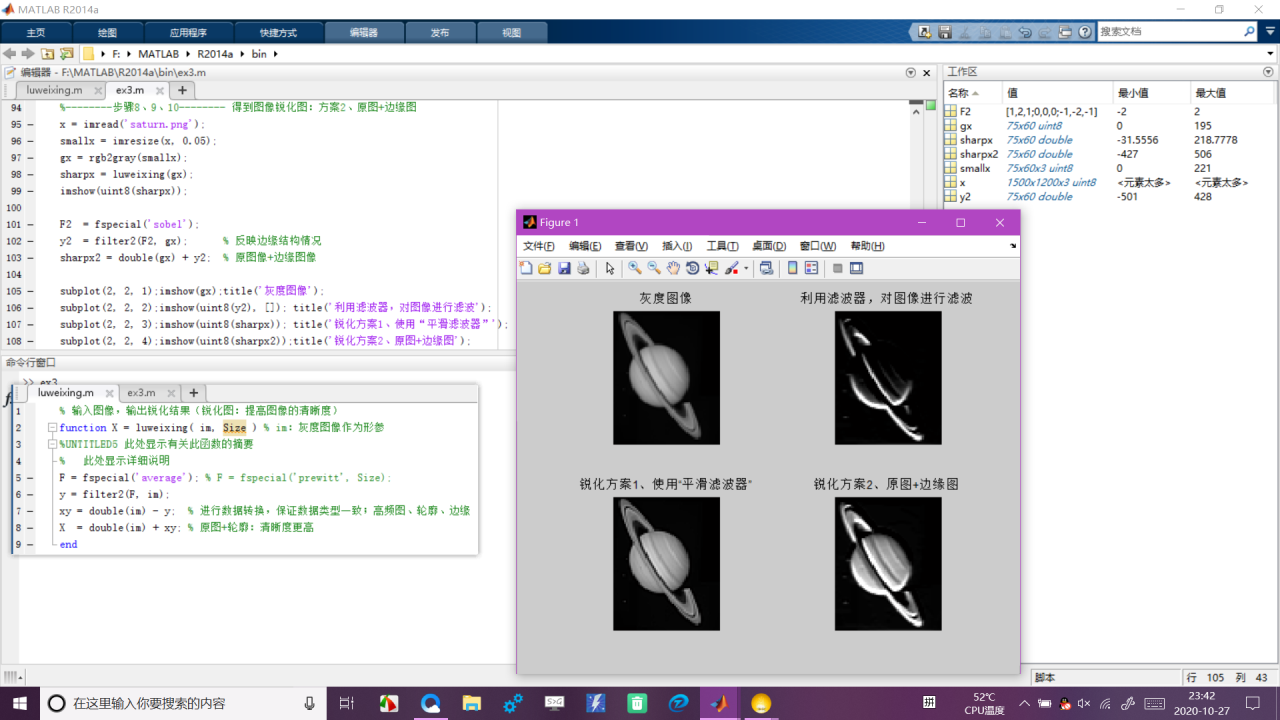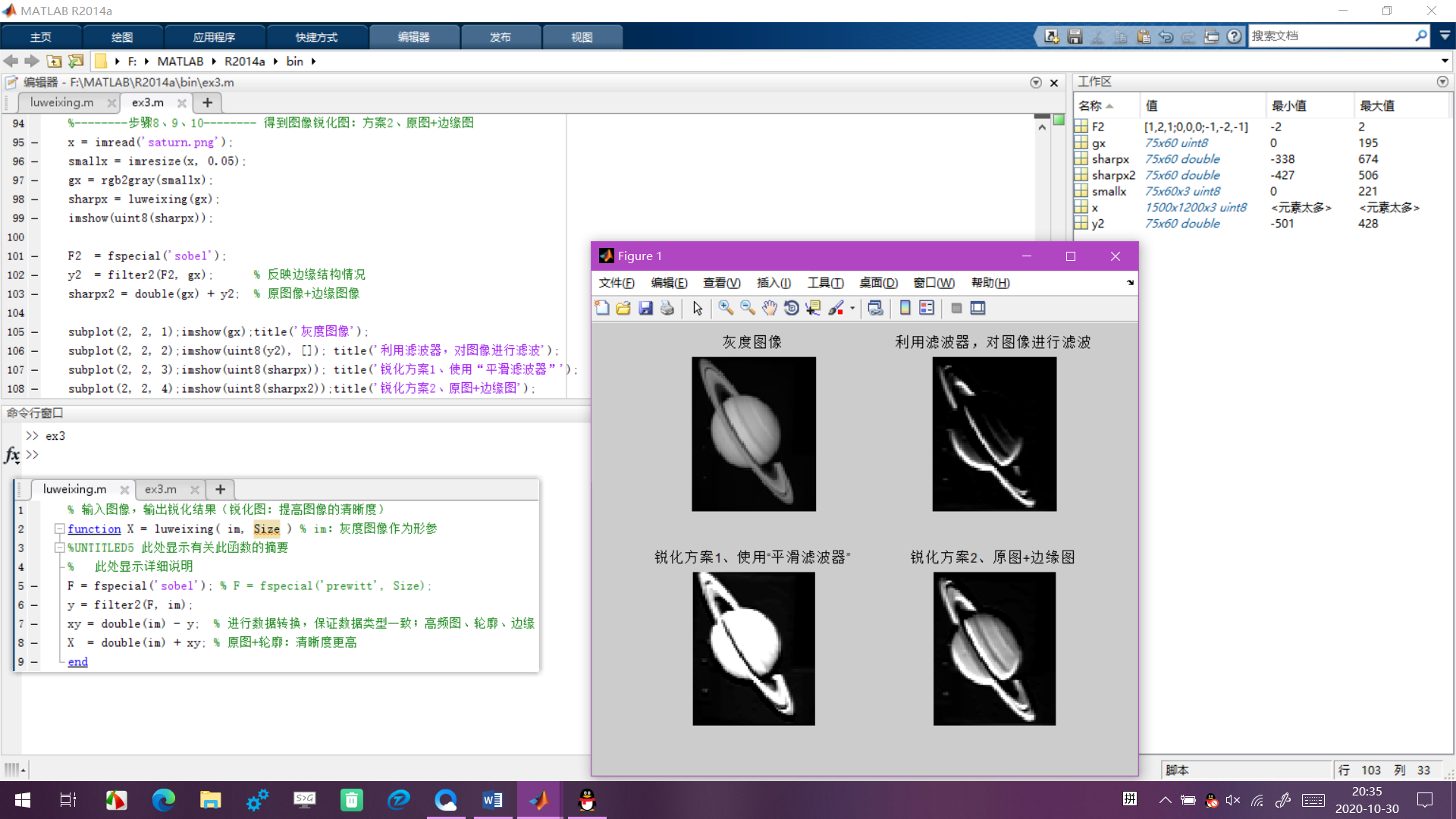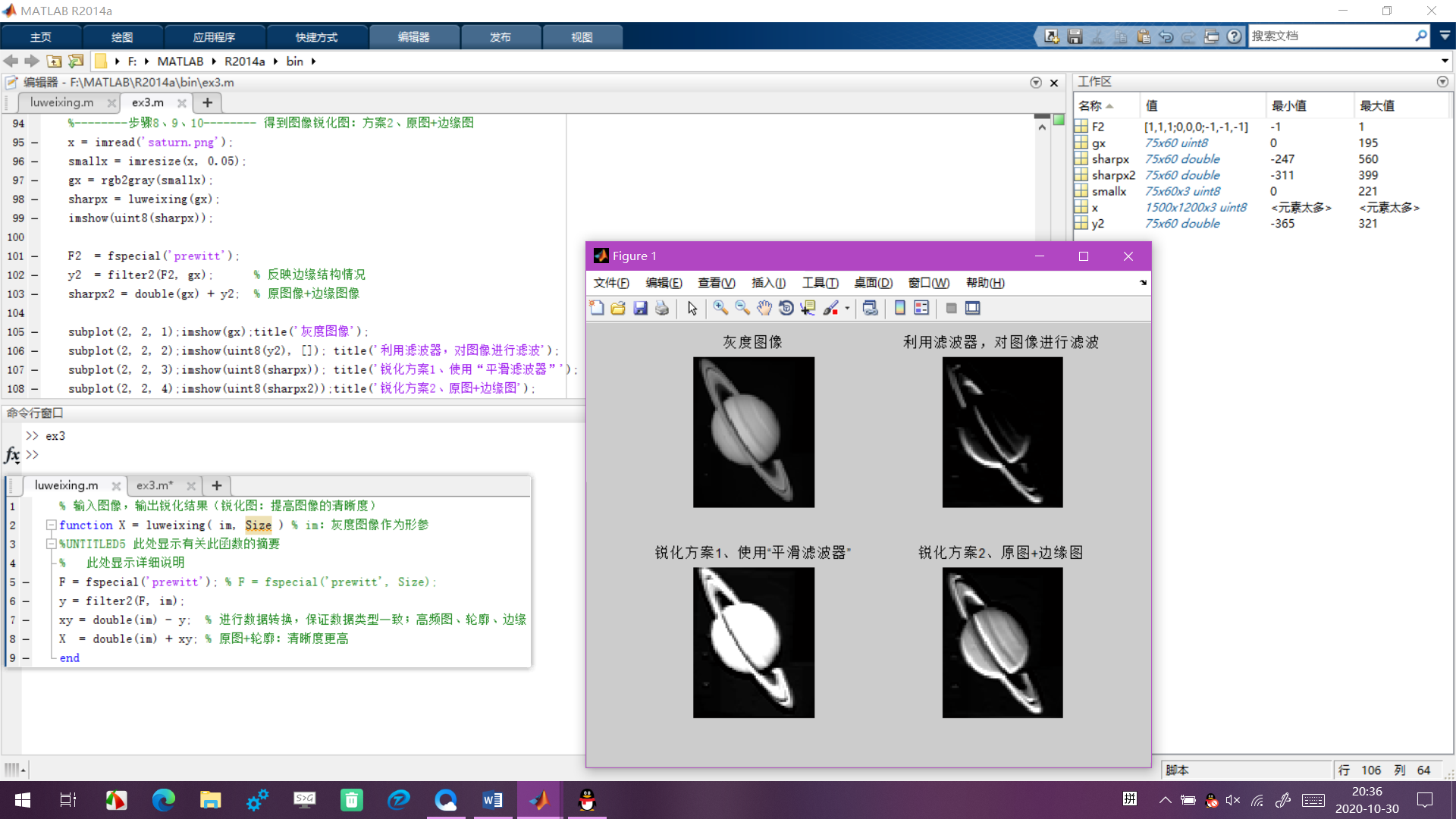四、分析与思考

【在此描述下你从该实验中得出的结论，并对实验结果进行分析，解释下锐化实现的过程和规律，例如滤波器参数的变化会导致锐化结果发生怎样的变化，可以查阅资料，该部分占最终成绩的50%】
此次实验，收获甚多，学会了Matlab中函数的使用。Matlab中函数的使用较为灵活，可以传递多个形式参数且形式参数的可使用数据类型比较多。使用函数，应尽可能地令函数功能最小化，一个函数不要实现太多的功能。
锐化图像的实现过程1：
首先利用grb2gray()函数将图像转换为灰度图像；然后使用边缘滤波器对灰度图像进行滤波（均值滤波器与高斯滤波器对图像进行滤波的效果较好），得到灰度图像的较为模糊的平滑图像；使用原图减去滤波结果图，得到突出边缘轮廓的高频图；最后，将原图加上突出边缘轮廓的高频图，即可得到锐化结果图。观察锐化结果图可以看出：锐化后的图像轮廓比原图像的轮廓清晰且锐化结果图清晰度更高。
锐化图像的实现过程2：
首先利用grb2gray()函数将图像转换为灰度图像；然后使用边缘滤波器对灰度图像进行滤波（均值滤波器与高斯滤波器对图像进行滤波的效果较好），得到灰度图像的较为模糊的平滑图像；原图+滤波结果图，即可得到锐化结果图。

附录：代码汇总
主文件——ex3.m
%--------步骤3--------
x = imread('saturn.png'); % 读取图片：将图像数据读取到Matlab环境中 peppers.png：Matlab内置图片
imshow(x);                % 显示图片
% 警告: Image is too big to fit on screen; displaying at 8%
% > In imuitools\private\initSize at 71
%   In imshow at 282

%--------步骤3--------
smallx = imresize(x, 0.05); % 缩小图片
figure, imshow(smallx);     % figure：生成新的图片窗口，不覆盖原窗口

% --------步骤4--------
smallx = imresize(x, 0.05);
imshow(smallx);
gx = rgb2gray(smallx);
f1  = fspecial('average', 5);
gx1 = filter2(f1, gx);
figure, imshow(uint8(gx1));

% --------步骤5-------- 利用平滑滤波器，对图像进行滤波
smallx = imresize(x, 0.05);
gx = rgb2gray(smallx);

f1 = fspecial('average', [9 9]);  % 1、9*9均值滤波器
gx1 = filter2(f1, gx);
f2 = fspecial('gaussian', [9 9]); % 2、高斯滤波器
gx2 = filter2(f2, gx);
f3 = fspecial('laplacian');       % 3、拉普拉斯滤波器
gx3 = filter2(f3, gx);
f4 = fspecial('sobel');           % 4、sobel滤波器
gx4 = filter2(f4, gx);
f5 = fspecial('prewitt');         % 5、prewitt滤波器
gx5 = filter2(f5, gx);

subplot(2, 3, 1);imshow(smallx);title('缩小至原来的0.05倍');
subplot(2, 3, 2);imshow(uint8(gx1));title('1、9*9均值滤波器');
subplot(2, 3, 3);imshow(uint8(gx2));title('2、9*9高斯滤波器');
subplot(2, 3, 4);imshow(uint8(gx3));title('3、拉普拉斯滤波器');
subplot(2, 3, 5);imshow(uint8(gx4));title('4、sobel滤波器');
subplot(2, 3, 6);imshow(uint8(gx5));title('5、prewitt滤波器');

% --------步骤5-------- 利用平滑滤波器，对图像进行滤波
smallx = imresize(x, 0.05);
imshow(smallx);
gx = rgb2gray(smallx);

f1  = fspecial('average', 5); % 1、均值滤波器
gx1 = filter2(f1, gx);
f2  = fspecial('gaussian');   % 2、高斯滤波器
gx2 = filter2(f2, gx);
f3  = fspecial('laplacian');  % 3、拉普拉斯滤波器
gx3 = filter2(f3, gx);
f4  = fspecial('sobel');      % 4、sobel滤波器
gx4 = filter2(f4, gx);
f5  = fspecial('prewitt');    % 5、prewitt滤波器
gx5 = filter2(f5, gx);
f6  = fspecial('disk');       % 6、disk滤波器
gx6 = filter2(f6, gx);
f7  = fspecial('log');        % 7、log滤波器
gx7 = filter2(f7, gx);
f8  = fspecial('motion');     % 8、motion滤波器
gx8 = filter2(f8, gx);
subplot(3, 3, 1);imshow(smallx);title('缩小至原来的0.05倍');
subplot(3, 3, 2);imshow(uint8(gx1));title('1、均值滤波器');
subplot(3, 3, 3);imshow(uint8(gx2));title('2、高斯滤波器');
subplot(3, 3, 4);imshow(uint8(gx3));title('3、拉普拉斯滤波器');
subplot(3, 3, 5);imshow(uint8(gx4));title('4、sobel滤波器');
subplot(3, 3, 6);imshow(uint8(gx5));title('5、prewitt滤波器');
subplot(3, 3, 7);imshow(uint8(gx6));title('6、disk滤波器');
subplot(3, 3, 8);imshow(uint8(gx7));title('7、log滤波器');
subplot(3, 3, 9);imshow(uint8(gx8));title('8、motion滤波器');

%--------步骤6、7-------- 得到图像锐化图：方案1、使用“平滑滤波器”
smallx = imresize(x, 0.05);
gx = rgb2gray(smallx);
sharpx = luweixing(gx, 5);

F  = fspecial('average', [9 9]); % 1、9*9均值滤波器
y  = filter2(F, gx);
xy = double(gx) - y;  % 进行数据转换，保证数据类型一致；高频图、轮廓、边缘
subplot(2, 2, 1);imshow(gx);title('灰度图像');
subplot(2, 2, 2);imshow(uint8(y),  []);title('1、利用滤波器，对图像进行滤波');
subplot(2, 2, 3);imshow(uint8(xy), []);title('2、高频图，突出边缘轮廓');
subplot(2, 2, 4);imshow(uint8(sharpx));title('3、锐化结果图');

%--------步骤8、9、10-------- 得到图像锐化图：方案2、原图+边缘图
smallx = imresize(x, 0.05);
gx = rgb2gray(smallx);
sharpx = luweixing(gx);
imshow(uint8(sharpx));

F2  = fspecial('prewitt');
y2  = filter2(F2, gx);      % 反映边缘结构情况
sharpx2 = double(gx) + y2;  % 原图像+边缘图像

subplot(2, 2, 1);imshow(gx);title('灰度图像');
subplot(2, 2, 2);imshow(uint8(y2), []); title('利用滤波器，对图像进行滤波');
subplot(2, 2, 3);imshow(uint8(sharpx)); title('锐化方案1、使用“平滑滤波器”');
subplot(2, 2, 4);imshow(uint8(sharpx2));title('锐化方案2、原图+边缘图');
函数文件
% 输入图像，输出锐化结果（锐化图：提高图像的清晰度）
function X = luweixing( im, Size ) % im：灰度图像作为形参
%UNTITLED5 此处显示有关此函数的摘要
%   此处显示详细说明
F = fspecial('prewitt'); % F = fspecial('prewitt', Size);
y = filter2(F, im);
xy = double(im) - y;  % 进行数据转换，保证数据类型一致；高频图、轮廓、边缘
X  = double(im) + xy; % 原图+轮廓：清晰度更高
end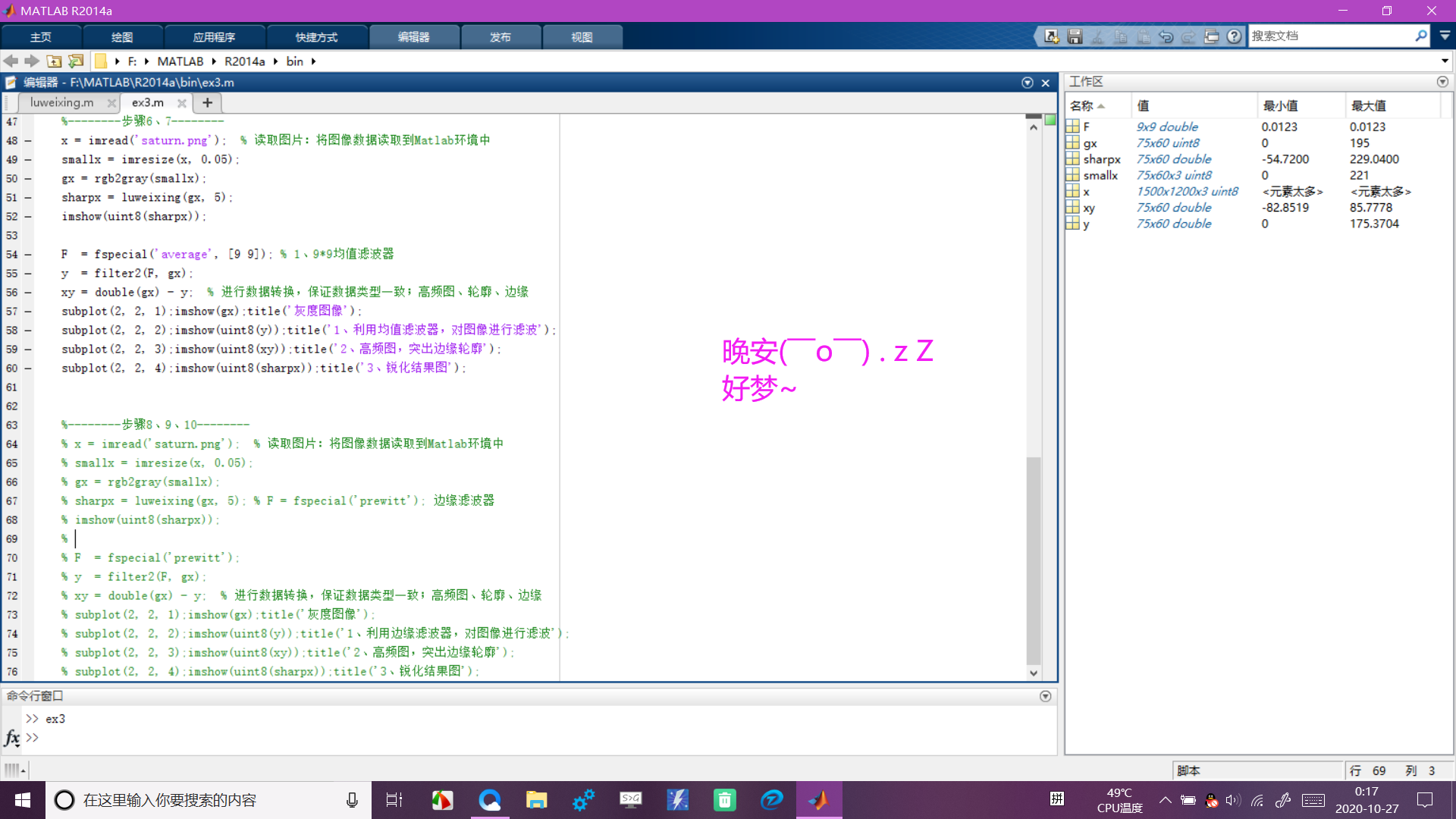展开全文计算机视觉 轮廓
• MATLAB图像处理 图书作者，代码从业者，N多年 ...
• 笔者阐述了一种基于MATLAB的数字图像处理系统设计，其中包括图像处理领域的大部分算法，运用 MATLAB图像处理工具箱对算法进行了实现，论述了利用系统进行图像显示、图形表换及图像处理过程，系统支持索引图像、...python java 大数据 数据分析
• 目录 图像反色 图像线性点运算 ...Matlab本擅长矩阵运算可以直接运算，由于实验要求不能使用矩阵运算，使用循环完成，以下其他点运算也是一样的处理，不再解释。 function OutImage = InverseImageCo...点运算 直方图均衡化 线性运算
• OpenCV3.2 Java图像处理视频培训课程：基于OpenCV新版本3.2.0详细讲述Java OpenCV图像处理部分内容，包括Mat对象使用、图像读写、 基于常用核心API讲述基本原理、使用方法、参数、代码演示、图像处理思路与流程讲授...计算机视觉 计算机视觉的研究方向 计算机视觉需要学什么
• ## matlab图像处理关于细胞计数

万次阅读 多人点赞 2018-02-02 12:13:40
在数字图像处理中，二值图像占有非常重要的地位，特别是在实用的图像处理中，以二值图像处理实现而构成的系统是很多的，要进行二值图像的处理与分析，首先要把灰度图像二值化，得到二值化图像，这样子有利于在对图像...
• ## MATLAB|数字图像处理

千次阅读 2021-01-03 14:00:45
MATLAB是一个强大的软件，今天给大家分享一下如何不用函数（如im2bw）实现彩图转灰度图、二值图以及给图片加上绿色的滤镜！欢迎大家点赞、讨论！
• 安装MinGW-w64 C/C++ 编译器 方法一： 下载地址：... 方法二： MATLAB Support for MinGW-w64 C/C++ Compiler： https://www.mathworks.com/support/requirements/previous-releases.html https://www.mathwlabview
• 本篇内容是我用matlab学习图像处理的记录，第一部分总结了matlab基础语法；第二部分实现了一个图像处理入门的算法。边缘检测 直方图
• 本文为芬兰米凯利应用科学大学（作者：Justyna Inglot）的信息技术学士论文，共65页。附录包含所有的MATLAB源...本文所提出的解决方案为图像处理未来的发展打开了一扇大门，并在最后一章阐述了实现的可能性及具体思路
• ## 基于MATLAB的语音信号处理

万次阅读 多人点赞 2018-07-15 01:21:20
基于MATLAB的语音信号处理摘要：语音信号处理是目前发展最为迅速的信息科学研究领域中的一个，是目前极为活跃和热门的研究领域，其研究成果具有重要的学术及应用价值。语音信号处理的研究，对于机器语言、语音识别、...
• MATLAB图像处理 精通c++，网络编程，数字图像处理领域，研究方向为数学...
• 本文用matlab实现了基本多边形的检测、提取。  本文涉及到的知识点如下：  1、Canny边缘检测。 bw = edge(gray,'canny',[0 , 50/256]);  2、细化操作。 im=bwmorph(image,'thin',Inf);  3、边界追踪。 edge...边缘检测 多边形 识别
• 带你玩转MATLAB图像处理 图像和算法等领域有多年研究和项目经验；指导发表...
• matlab做遥感数字图像处理（指导老师：蔡国林）
• 数字图像处理实验 文章目录数字图像处理实验前言一、实验目的和要求：二、实验所用函数三、实验结果1、图像的傅里叶变换2、频谱和相位谱重要性的验证 前言 本文对图像进行傅里叶变换、显示频谱、相位谱等操作；并且...
• 读入一幅彩色图像，进行如下图像处理： 1. 在RGB颜色空间中对图像进行平滑滤波（模糊）和锐化滤波（锐化）处理。 1.1. 读入一幅图像。 rgb = imread('flower.jpg'); figure; imshow(rgb); title('原图...
• 基于MATLAB图像融合设计摘 要图像融合能够将不同类型传感器获取的同一对象的图像数据进行空间配准。并且采用一定的算法将不同类型的传感器获取的同一对象的图像数据所含用的信息优势或互补性有机地结合起来产生的...算法 大数据 python 计算机视觉
• 使用Fourier变换把图像从空间域变换到频域，在频域内做相应增强处理，再从频域变换到空间域得到处理后的图像,我们这里主要学习Fourier变换和FFT变换的算法.傅立叶变换 图像傅立叶变换
• 前言：作为一名图像算法攻城狮，那是在2014年大三下学期，一本绿色版的《数字图像处理》（冈萨雷斯的英文版）出现在自己课桌前。偶然间打开的一扇意外之门，就这样结下了一段不解之缘，那些日子不断上网搜代码的自己...计算机视觉 课堂作业
• 前言：作为一名图像算法攻城狮，那是在2014年大三...通过本实验使学生掌握用MATLAB进行数字图像处理的基本语句。 二、实验原理 本实验室基于数字图像处理课程中的数字图像基本概念来设计的。 本实验的准备知识：MATLAB矩阵 经验分享 计算机视觉
• 图像进行拉普拉斯变换的matlab程序,对初学者了解拉普拉斯变换很有帮助
• 3.于MATLAB环境下编程实现对图片锐化。三、实验内容 利用图像基于单方向梯度算子，Robert算子，Sobel算 子，Pritt算 子, Laplacian算子，多方向模板，LoG算子编写matlab增强程序;最后，显示边缘图像和锐化增强图像，...
• 将搜索到的谷底对应的灰度值作为灰度阈值，并对大于或小于灰度阈值的部分作相应的处理图像中所有灰度值大于阈值的像素点认为是组成物体的点，称为目标点；将图像中那些灰度值小于等于阈值的像素点认为是组成...阈值分割...

# matlab图像处理编程思路matlab 订阅